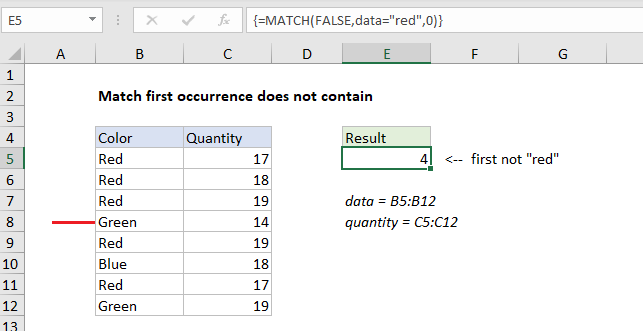## Excel Office

Excel How Tos, Tutorials, Tips & Tricks, Shortcuts

# Match first occurrence does not contain

This tutorial shows how to Match first occurrence does not contain in Excel using the example below;

## Formula

`{=MATCH(FALSE,logical_test,0)}`## Explanation

To get the position of the first match that does not contain a specific value, you can use an array formula based on the MATCH, SEARCH, and ISNUMBER functions. In the example shown, the formula in E5 is:

`{=MATCH(FALSE,data="red",0)}`

where “data” is the named range B5″B12.

Worked Example:   Merge tables with VLOOKUP in Excel

Note: this is an array formula and must be entered with control + shift + enter.

### How this formula works

This formula depends on a TRUE or FALSE result from a logical test, where FALSE represents the value you are looking for. In the example, the logical test is data=”red”, entered as the lookup_array argument in the MATCH function:

`=MATCH(FALSE,data="red",0)`

Once the test is run, it returns an array or TRUE and FALSE values:

`=MATCH(FALSE,{TRUE;TRUE;TRUE;FALSE;TRUE;FALSE;TRUE;FALSE},0)`

With the lookup_value set to FALSE, and match_type set to zero to force and exact match, the MATCH function returns 4, the position of the first FALSE in the array.

Worked Example:   How to check cell that contains one of many with exclusions in Excel

### Get associated value

To retrieve the associated value from the Quantity column, where “quantity” is the named range C5:C12, you can use INDEX and MATCH together:

`{=INDEX(quantity,MATCH(FALSE,data="red",0))}`

### Literal contains

If you need to match the first value that literally “does not contain”, you can use a variant of the formula. For example to match the first value in data that does not contain an “r”, you can use:

`=MATCH(FALSE,ISNUMBER(SEARCH("r",data)),0)`
Worked Example:   Two-way lookup with INDEX and MATCH in Excel